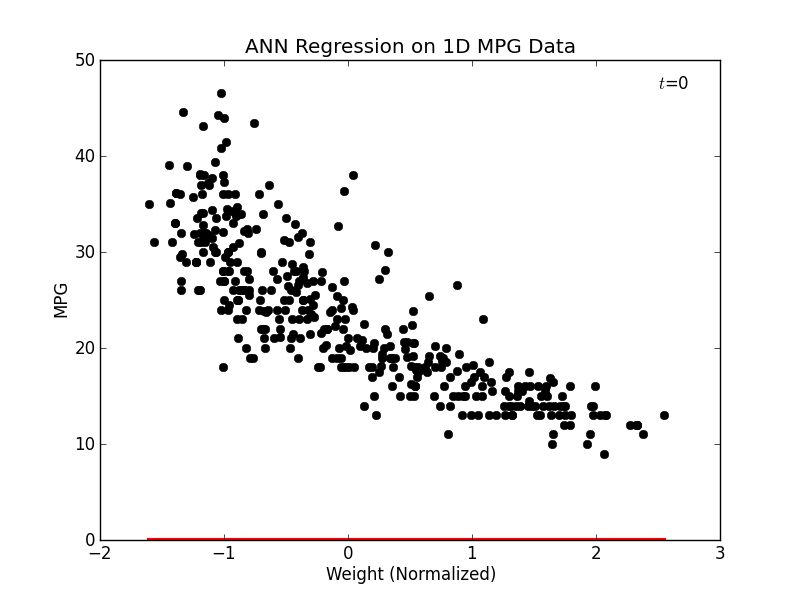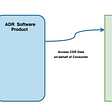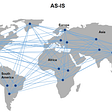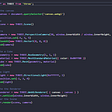# Generating things with code

## part 2 of 2: process and systems

In the first part of this post, we explored the nature of generative systems, we emphasized the importance of data and space in generative design and highlighted the process as a third component.

## THE ELEPHANT IN THE ROOM

before we start, I need to disambiguate what will follow from a field that may seem identical: data-visualization. Data-visualization is the field of illustrating — illustrate literally means ‘bring to light’ — a data-set. I won’t go too much into details as it’s not my field of expertise, but there are two main types of visualizations: informative and exploratory. Informative visualizations are meant to give an insightful representation of a data-set, exploratory visualizations on the other hand are more of a tool that allows the user to make sense of a data-set.

Generative design is closer to the latter and the main difference between a data-visualization and a generative system outcome is that the data-set is often produced by the generative system while the data-visualization should not alter its source material. If we look back at our previous examples, No Man’s Sky and the Kinematic dress are systems that generate their own data while the unnamed soundsculpture, could be considered an exploratory data-visualization where the camera can choose arbitrary points of view on a pre-recorded data-set.

In the first two examples, the simulation allows the user to alter the model (destroying a rock in No man’s sky changes the state of the game and changing the initial Kinematic dress model will change the outcome of the simulation) while in the third example, the source material — a 3d point cloud recorded over time — is never altered by the changes made in the camera position.

Though it’s not the point of this article, data-visualization is an amazing discipline and I would really encourage anyone to explore it further.

# PROCESS

We saw the intricate relationship that data maintain with their space of representation and vice versa. A process can be thought of as data-space design. It’s a field with few canonical rules and where creativity arises.

## DISTRIBUTION

The first thing we want to do when we found an interesting data-set is to view it in space. This process can be referred to as a distribution ; the fact of displaying our data in space in a plain and legible way. Distribution is a N-dimensional process but I find more intuitive to explain it it 2D so, to keep things simple, we’ll work with 2D data. The example below uses translations to distribute 2D cartesian data points (X/Y coordinates), on a 2D canvas.

We have a regular grid that covers the whole 2D space and I have added conditions to isolate some areas of this regular grid as a sub grid and as a frame.

As seen in the previous article, 2D data points can also represent polar data: an angle and a radius. The following example introduces the notion of rotation and illustrates how to place points on a 2D canvas based on an angle and a radius.

First we have a ring of points: a fixed angle and a fixed radius, then a series of discs drawn at a fixed angle with an increasing radius and finally increasing both the angle and the radius gives us a spiral.

The third distribution method is the scale, it allows to resize a data point in space. This example shows a regular polygon being progressively rescaled.

Scaling allows us to ‘fit’ large data-sets into a small space or emphasize some data points depending on one of their dimensions. The scaling does not have to be the same of the various dimensions of our objects in which case it’s called skewing. It’s worth noting that rotations and scales are always relative to an origin. It is therefore quite common to first translate the data to the origin, preform the scaling and rotation before translating the data points back where they were originally.

It is of course possible to distribute data in other ways, for instance this ‘triangular’ or ‘hexagonal’ grid is no more than a regular grid where cell sizes are computed from an equilateral triangles’ dimensions.

These three simple methods are often bundled together under the acronym PRS for Position/Rotation/Scale, they are essential to manipulate data-spaces and offer many variations, for instance this snippet:

`var golden_angle = Math.PI * 2 / ( ( 1 + Math.sqrt(5) ) / 2 );var count  = 1024;for( var i = 0; i < count; i++ ) {    var theta = i * golden_angle;    var radius = Math.sqrt( i / count ) * h / 3;    circle(Math.cos(theta) * radius,Math.sin(theta) * radius, 5 );    circle(Math.cos(theta) * radius,Math.sin(theta) * radius, 1 );}`

gives this ‘sunflower seeds’ distribution:

## LERP, NORM, MAP

When it comes to distribution, I can’t think of anything more useful than these 3 methods:

`function lerp ( t, a, b ){    return a * (1-t) + b * t;}function norm( t, a, b ){    return ( t - a ) / ( b - a );}function map( t, a0, b0, a1, b1 ){    return lerp( norm( t, a0, b0 ), a1, b1 );}`

Lerp stands for linear interpolation, it returns a blend between 2 values `a`and `b` given a value `t` between 0 and 1. Norm means normalization, it will return a value in the range [0-1] given a value `t` comprised between `a` and `b` . Finally, the map method will combine lerp and norm to obtain the interpolated value of the normalized ratio of `t` between `a0-b0`, over the range `a1-b1`.

These `lerp` and `norm` are implemented in many APIs though their name and signatures may vary. In GLSL, lerp is called `mix(a,b,t)` and norm is called `smoothstep(a,b,t)` the `map` method is rarely there by default but as it is a combinatorics of the other two, it’s straightforward to implement.

As mentioned above, I find 2D distributions easier to understand but it is important to remember that they are N-dimensional, so they can work in 1, 2, 3, 4.. dimensions. What happens in the range [0-1] deserves a full article.

## TRANSFORMATION

Once the data are distributed in space, the next process we can think of — as we’re interested in shapes’ generation — is the transformations ; literally the fact of turning something into something different. To tackle this new concept, we will need to be able to compute relations between our data points, namely angles and distances. Assuming we have data points with `x` and `y` properties, the angle and distance methods look like this in pretty much any language:

`//angle from point a to point bfunction getAngle(a,b) {    var dx = b.x - a.x;    var dy = b.y - a.y;    return Math.atan2(dy, dx);}//distance from point a to point bfunction getDistance(a,b) {    var dx = a.x - b.x;    var dy = a.y - b.y;    return Math.sqrt(dx*dx + dy*dy);}`

As common as they may seem, these 2 methods are involved in pretty much any generative system, the most famous of which is probably the node garden but Boids, attraction repulsion, particle engines, stippling, disc packing often don’t need more than the angle/distance methods.

For convenience, the following examples will use a `Point` object to hold the `x` and `y` values. Let’s start with a second take at rotations. In the following example, we distribute points along a jagged line and perform a rotation of the points around an arbitrary center (usually called a lattice):

We could have used a regular polar distribution: at each step along the line, we would store the angles/radius of the point, then we would redraw the series of segments with an increasing start angle. It would work for simple shapes but if we had a complex object instead of a simple jagged line, it would be much easier to use this method to transform the point set.

In the previous article, we saw that in order to reduce the number of dimensions of an object, we often need to project it onto a space with fewer dimensions. The following example illustrates this principle as our 2D data points are being projected onto a line (1D).

Projections are massively used in game engines as well as for their aesthetics qualities, it’s a sort N-dimensional ‘primitive’ method. For instance reflecting the data about an axis uses the projection method then translates the result.

A kaleidoscope will only use points symmetry (rotation transform) and reflections. The example above highlights the fact that there is no need to work with complex data to get a complex outcome ; combining the transformations help us create complexity from series of simple processes, enter the systems.

## RULE-BASED SYSTEMS

Most tesselations of the plane (point symmetries, friezes and pavings) use only the above methods in a specific order to achieve complex looking patterns. By systems I mean the organization of processes to achieve a result, in this sense it is very close to an algorithm. Just like the distribution of data in space requires some simple methods, designing a system also requires some tools the most fundamental of which is probably a grammar.

A grammar is a series of rules that defines all the valid sentences of a language. As we’re mostly concerned with shape’s generation, we can extend this grammar to what is known as a formal grammar ; a system based on production rules, that takes an axiom as an input and determines if it is valid. The next illustration is a ‘finite state machine’ (FSM) that describes all the production rules relationships of a formal grammar:

It may seem complex and a bit cryptic but the interesting thing here is that instead of writing a fixed dictionary of all the valid solutions and check our axiom (input value) against all the dictionary’s entries, we can use this small system to check if any axiom is valid:

# L-SYSTEM

I said earlier that there are few canonical rules when dealing with processes, there is one seminal system though and it sprung a constellation of generative systems: the L-system. The ‘L’ stands for ‘Lindenmayer’, a Hungarian biologist who wanted to model plants’ growth in a compact way and came up with this notation. L-systems are a variation on formal grammar, if we set the following production rules:

`rules  : (A → AB), (B → A)`

and use`A` as an axiom, the system will produces the following results:

`n=0:         A           start (axiom/initiator)            / \n=1:       A   B         (A→AB)          /|    \n=2:     A B     A       (A→AB), (B→A)        /| |     |\n=3:   A B A     A B     (A→AB), (B→A), (A→AB)      /| | |\    |\ \n=4: A B A A B   A B A   (A→AB), (B→A), (A→AB), (A→AB), (B→A)`

With an axiom (`A`) and a ruleset (`(A → AB), (B → A)`), we iterate for a given number of generations (`n` ) and create a new axiom by reading each letter and replacing the occurrences of the variables ( `A & B` ) using the corresponding rules. Then we feed the axiom back into the system until we reach the desired iteration count. Note how, after 4 generations, we obtained a tree like structure.

This could have remained a confidential scientific notation but the L-systems can easily be turned into a graphics representation. In its graphic form, we use a turtle (a vector cursor) and the rule set controls its position, orientation and stride. Here’s a demo, try to change the axiom and the rewriting rule, using `f, +, -` and `[]` to create branches.

L-systems quietly introduced the concepts of: iteration, feedback, rewriting, turtle, recursion, graph & emergence. As disappointing as it may sound, there is not much more to generative systems, let’s explore each of these components.

## ITERATION

This principle is probably the simplest to understand if you’ve used a computer already, I used it in almost all the examples so far, it’s the `for` and `while`loops. These statements allow a process to run for a given amount of times or until a condition is met (respectively). Loops will let us apply a process to arrays of objects instead of dealing with individual entities. In the previous examples, I would iterate an angle variable from 0 to PI*2 and use the current iteration value as the angle at which I wanted to place a dot.

## FEEDBACK

This is also quite intuitive if you’ve ever animated something with code ; it’s the fact of using the previous state of an object to compute the next. if you want to move a dot from left to right on a screen, you could write something like: `dot.x += value` and you would be using a feedback ; using the previous x position of the dot to compute the next.

here’s a simple example of an iterative process taking advantage of the previous state of the objects:

some points are being drawn at random on the screen, then their position is incremented using a polar transform (angle/radius) for a given amount of iterations (100).

## REWRITING

The fact that L-system uses a feedback rather than an instantaneous process allows to read the current state of an object, alter it and only then update (rewrite) the whole object rather than some of its properties. This two-step process is not frequently used but it is very powerful, image filters use it a lot.

## TURTLE

In its graphic form, the L-system uses a turtle, it’s the most common form of ‘brush’ or ‘pencil’ when using a graphic API. A turtle can move forward (draw a line), rotate left and right in place and teleport to any point of the canvas. In modern versions, it can also save a state (position/rotation), do something and come back to the saved state. In more recent generative systems, turtles are also called agents ; an augmented data-holder, generally semi-autonomous, that does more than a regular turtle but remains essentially a way of drawing things on screen.

## RECURSION

It’s the fact of applying the same process until an exit condition is met. Unlike iterations, the recursive process will call itself repeatedly which makes it very tricky to master as you may well freeze your platform if your exit condition is never met. A canonical example of recursion is the sierpinski triangle where a triangle is recursively subdivided a given amount of times.

This is a very powerful tool and a rich example as it introduces the notion of self similarity (fractals, I’m looking at you).

## TREES and GRAPHS

Another notion introduced by the L-system was the graph. When we unfold the algorithm, generation after generation, a tree-like structure emerges. Trees are a special kind of graphs that are said to be acyclic as they have no loop (cycle) ; there is a single root node and branches cannot be connected to themselves or other branches. By design, a L-system can only produce trees but graphs are very handy data structures. Here’s a minimal Graph code:

`var Vertex = function( data ){ /*store the data*/ }var Edge = function( v0, v1 ){    this.v0 = v0;    this.v1 = v1;}var Graph = function( vertices, edges ){    this.vertices = vertices;    this.edges = edges;}//create two verticesvar A = new Vertex( data );var B = new Vertex( data );//create an edge to bind the two verticesvar E = new Edge( A, B );//create a graphvar graph = new Graph( [ A, B ], [ E ] );`

One of the (many) features of this data structure is that vertices can be updated independently from the edges. If you’re interested, I wrote a longer article about this topic.

## EMERGENCE

Last but not least, the L-system exhibits an emergent behavior. This is the case when you build a system where “larger entities arise through interactions among smaller or simpler entities such that the larger entities exhibit properties the smaller/simpler entities do not exhibit.” (wikipedia). Indeed, when you craft the ruleset and feed the axiom, there is no telling what the resulting outcome will be.

I’ll leave you with this example of a flocking system to illustrate this principle.

Emergence is doubtlessly the most beautiful property of generative systems and what most artists are after. If there was but one thing to remember from this article, it would be that complexity arises from a sum of simplicities.

The above example uses only translations & a distance function.

--

--

--

graphic coder

Love podcasts or audiobooks? Learn on the go with our new app.

## Recommended from Medium## “Industry knowledge is a great advantage for Data Scientists”## A peek into classic Auto MPG Dataset and DNN model to predict the fuel efficiency of the late-1970s…## Top 3 Frustrations In Preparing Data For Analysis## Bubble Sort: Implementation and Analysis## Consumer Data Right (CDR)— Consent and it’s characteristics!!## [Worker Proposal] WARP Network을 제안합니다 — Part 1## Extracting Data from a Spider Graphgraphic coder

## Fast way to animate 3D model using PixCap and ReadyPlayerMe## ThreeJS — Adding the Orbit Controls## How to create ‘Component/Library’ in ESP-IDF for ESP32## DALL-E2 is amazing for creating images from text.